# Math in Focus Grade 6 Chapter 1 Lesson 1.2 Answer Key Prime Factorization

Go through the Math in Focus Grade 6 Workbook Answer Key Chapter 1 Lesson 1.2 Prime Factorization to finish your assignments.

## Math in Focus Grade 6 Course 1 A Chapter 1 Lesson 1.2 Answer Key Prime Factorization

### Math in Focus Grade 6 Chapter 1 Lesson 1.2 Guided Practice Answer Key

Complete.

Question 1.
Express 48 as a product of its prime factors.
Method 1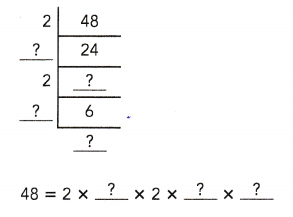Method 2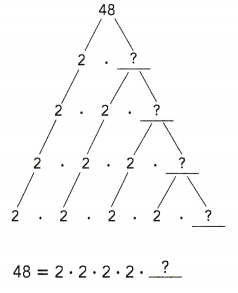### Math in Focus Course 1A Practice 1.2 Answer Key

Question 1.
Copy the array of numbers. Circle all the prime numbers.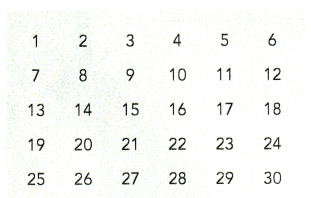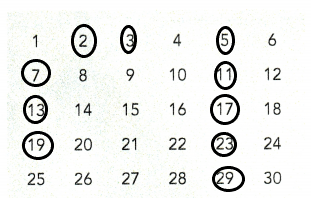Explanation:
I circled all the prime numbers between 1 and 30.

Express each number as a product of its prime factors.

Question 2.
6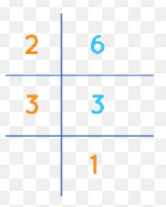6 = 2 x 3

Explanation:
2 and 3 are the prime factors of 6.

Question 3.
15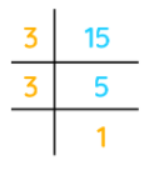15 = 3 x 5

Explanation:
3 and 5 are the prime factors of 15.

Question 4.
36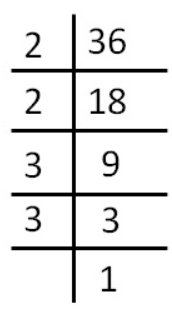36 = 2 x 2 x 3 x 3

Explanation:
2 and 3 are the prime factors of 36.

Question 5.
78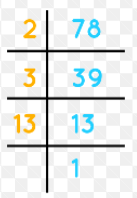78 = 2 x 3 x 13

Explanation:
2, 3 and 13 are the prime factors of 78.

Question 6.
184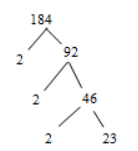184 = 2 x 2 x 2 x 23

Explanation:
2 and 23 are the prime factors of 184.

Question 7.
360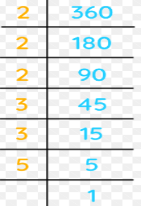360 = 2 x 2 x 2 x 3 x 3 x 5

Explanation:
2, 3 and 5 are the prime factors of 360.

Question 8.
24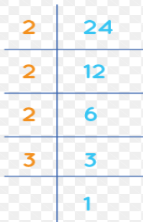24 = 2 x 2 x 2 x 3

Explanation:
2 and 3 are the prime factors of 24.

Question 9.
49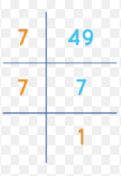49 = 7 x 7

Explanation:
7 is the only prime factor of 49.

Question 10.
81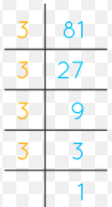81 = 3 x 3 x 3 x 3

Explanation:
3 is the prime factor of 81.

Question 11.
144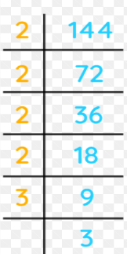144 = 2 x 2 x 2 x 2 x 3 x 3

Explanation:
2 and 3 are the prime factors of 144.

Question 12.
245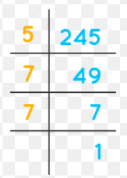245 = 5 x 7 x 7

Explanation:
5 and 7 are the prime factors of 245.

Question 13.
510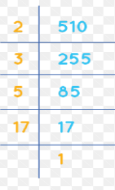510 = 2 x 3 x 5 x 17

Explanation:
2, 3, 5 and 17 are the prime factors of 510.

Question 14.
250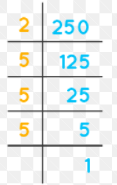250 = 2 x 5 x 5 x 5

Explanation:
2 and 5 are the prime factors of 250.

Question 15.
1,089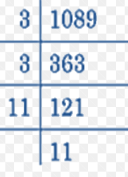1089 = 3 x 3 x 11 x 11

Explanation:
3 and 11 are the prime factors of 1089.

Question 16.
4,725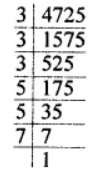4725 = 3 x 3 x 3 x 5 x 5 x 7.

Explanation:
3, 5 and 7 are the prime factors of 4725.

Question 17.
900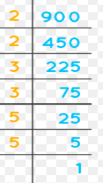900 = 2x 2 x 3 x 3 x 5 x 5

Explanation:
2, 3 and 5 are the prime factors of 900.

Question 18.
27,000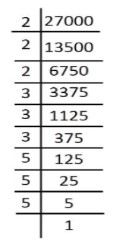27000 = 2x 2 x 2 x 3 x 3 x 3 x 5 x 5 x 5.

Explanation:
2, 3 and 5 are the prime factors of 27000.

Solve.

Question 19.
Math Journal Describe the steps for finding the prime factors of 42.

Question 20.
400 written as a product of its prime factors is 2 × 2 × 2 × 2 × 5 × 5. Write 800 as a product of its prime factors.
2 x 2 × 2 × 2 × 2 × 5 × 5 = 800

Explanation:
800 written as a product of its prime factors is 2 x 2 × 2 × 2 × 2 × 5 × 5.

Question 21.
Given that 320 written as a product of its prime factors is 2 × 2 × 2 × 2 × 2 × 2 × 5, write 3,200 as a product of its prime factors.
2 x 2 × 2 × 2 × 2 × 2 × 2 × 5 x 5 = 3200

Explanation:
3200 written as a product of its prime factors is 2 x 2 × 2 × 2 × 2 × 2 × 2 × 5 x 5 .

Question 22.
2,700 written as a product of its prime factors is 2 × 2 × 3 × 3 × 3 × 5 × 5. Write 270 as a product of its prime factors.
2 × 3 × 3 × 3 × 5 = 270

Explanation:
270 written as a product of its prime factors is 2 ×  3 × 3 × 3 × 5.

Question 23.
It is given that 4,800 can be expressed in terms of its prime factors as 2 × 2 × 2 × 2 × 2 × 2 × 3 × 5 × 5.

a) Write 1,200 as a product of its prime factors.